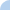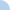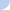# mcchpt07_quiz_b04_cp_form_mass_per_comp_emp_formula_2012_do-over

Answer the following with no units. Recall that we round all atomic masses to whole numbers except for Cl and Cu, for which we use 35.5 and 63.5, respectively. Only formulas with an odd number of Cl or Cu should have a decimal. (and these should have .5) You will need a periodic table. Determine the % by mass to 3 significant figures, i.e. - XX.X or X.XXQuiz Log In